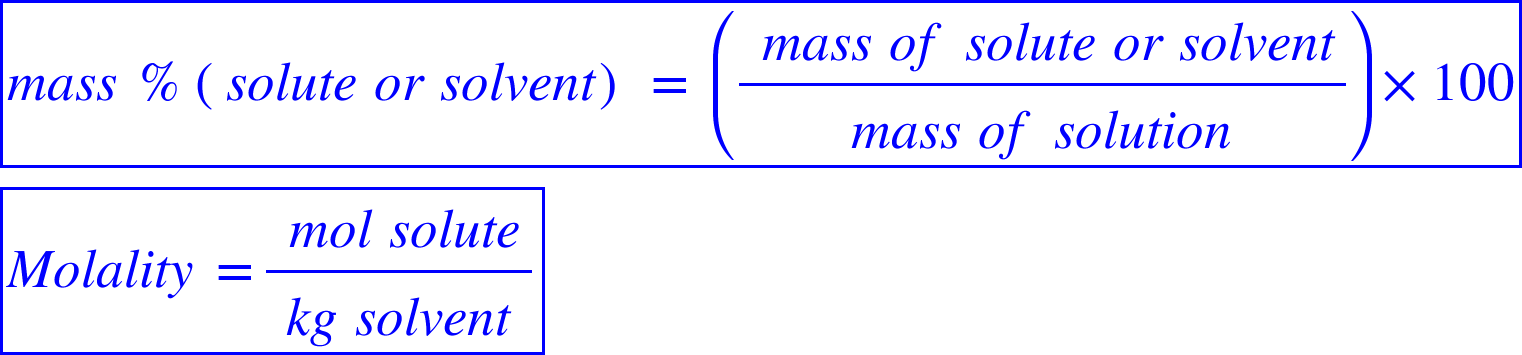# Problem: An aqueous solution contains 32% HCl by mass.Calculate the molality of the solution.

🤓 Based on our data, we think this question is relevant for Professor Sheagley's class at PDX.

###### FREE Expert Solution

We will start by defining the mass percentage and molality.We’ll solve this problem through these steps:

• Find the mass of water
• Find the molar mass of HCl
• Finding the moles of HCl
• Finding molality from mol HCl and mass of water###### Problem Details

An aqueous solution contains 32% HCl by mass.

Calculate the molality of the solution.